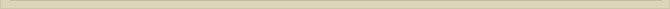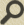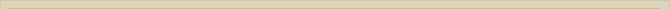When you are interested in physics you must read “Unbelievable“!

The energy quantification of the atoms at molecular distance are possibly the result of the quantification of distance.

In the book “From Paradox to Paradigm”, chapter “The photon and the constant of Planck” , the Planck-radius is calculated at: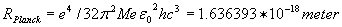(12);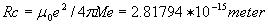(13)

We observe that the ratio between the Compton-radius and the Planck-distance is: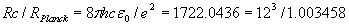exactly the same factor as between the rydberg radius and the Bohr-radius (11). ***

We will show that this equality is not just a coincidence, but the result of the existence of the quantum distance (QD). The Planck-distance, the Compton radius, the Bohr-radius and the rydberg constant are directly and integer related the quantum number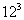.

The derivation of the Planck-distance is based on the assumption that space is not absolutely empty, but that space is filled with so called point-volumes with a radius of the Planck-distance. Although a not empty space is formally not consistent with the assumption of science that space is absolutely empty, science already admits inherently that space is not empty the general acceptation of the field theory. The field theory assumes that in “empty” space, vacuum, there can be fields such as electrostatic fields, magnetic fields and gravity fields.

How can there exist fields in vacuum when this vacuum is assumed to be absolutely empty?

Philosophically this is not considered possible. Science already implicitly accepts that vacuum is not absolute empty, only science doesn’t admit it yet officially or formally!

There are more very strong indications that vacuum is not just empty space. The phenomenon of stellar aberration for example indicates strongly that there is “ether” (“Stellar Aberration and the Unjustified Denial of Ether” Galilean Electrodynamics 16, 75-77 (July/August 2005)).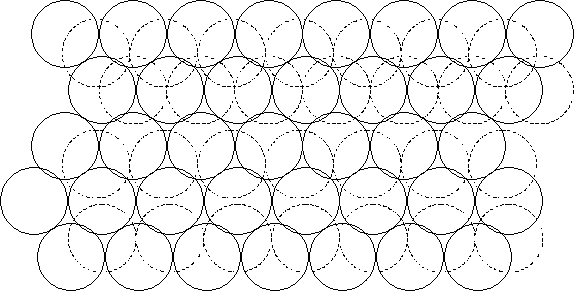Figure 2.  An impression of space filed with point-volumes.

So when we assume space is not empty but filled with so-called point-volumes this is scientifically not unacceptable. The remarkable thing that happens is that when we fill up space with point-volumes a completely QM-consistent explanation for the 12 atomic ionization levels is found and at the same time calculations of the correct distance/energy level of the nucleus at the ionization levels are obtained. Although mainstream science rejects ether, the scientific explanations are too compelling to ignore.

When we imagine that space is filled up with bulb shaped point-volumes with radius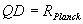then space cannot be homogeneous everywhere (figure 2).

Next chapter: The Orientation of Point-Volumes Surrounding a Charge